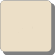#### Howdy, Stranger!

We are about to switch to a new forum software. Until then we have removed the registration on this forum.

# To anyone experiencing low frame rates when using image() many times

edited August 2017

Greetings,

I had a very big problem in an app I'm developing. So far I've only used it JAVA Mode, and my framerates where about 20-30 fps, so on a tablet the sketch would never work. I've googled to my best ability but couldn't find a solution.

The game is a word game, and has an 9*11 grid of letter tiles (plus the background). Each one of these is a pre-loaded PImage of 55x55px. If I remove the image() function in the tiles display() method, the problem went away.

So my question was how in the world do I display that many images without problem? A game like Minecraft can display thousands of images, in higher pixel dimensions, in 3D, and at high framerates. Why couldn't I?

I reduced the code to the problem below (image file below too if you want to test). Right before I was about to post on this forum to get help, I tried taking the size to display parameters out of the image() call. And it worked. The on-the-fly display size of the image was taking up way too much CPU.

If you're having this same problem, hopefully your problem is the same, and you can fix it easily like I have. Modifications:

Add this in setup: `tile.resize(round(full_tile), round(full_tile));`

Change this in draw: `image(tile, grid[x][y].x, grid[x][y].y);`

And that is it! Hope this helps!

``````PImage tile;

float full_tile;
float three_quarter_tile;
float half_tile;
float quarter_tile;
float eighth_tile;

PVector grid_tile_dims;
PVector[][] grid;
PVector grid_corner;

void setup()
{
size(1280, 720);

full_tile = height/12;
three_quarter_tile = full_tile * 0.75;
half_tile = full_tile * 0.5;
quarter_tile = full_tile * 0.25;
eighth_tile = full_tile * 0.125;

grid_tile_dims = new PVector(11, 9);
grid = new PVector[int(grid_tile_dims.x)][int(grid_tile_dims.y)];
float grid_step = eighth_tile;
grid_corner = new PVector(width - (half_tile + ((height - ((grid_tile_dims.y * full_tile) + ((grid_tile_dims.y - 1) * grid_step)))/2)), quarter_tile + ((height - ((grid_tile_dims.y * full_tile) + ((grid_tile_dims.y -1) * grid_step)))/2));

for   (int y = 0; y < int(grid_tile_dims.y); y++)
{
for (int x = 0; x < int(grid_tile_dims.x); x++)
{
float sep = 0;
if (x % 2 != 0.0) sep = half_tile;
grid[x][y] = new PVector(grid_corner.x - ((full_tile + grid_step) * x), sep + grid_corner.y + ((full_tile + grid_step) * y));
}
}
}

void draw()
{
background(255);

for (int y = 0; y < int(grid_tile_dims.y); y++)
{
for (int x = 0; x < int(grid_tile_dims.x); x++)
{
if(!mousePressed) image(tile, grid[x][y].x, grid[x][y].y, full_tile, full_tile);
}
}

fill(0);
textAlign(LEFT, TOP);
textSize(height/32);
text(int(frameRate), 2, 2);
}
``````Tagged:

• Excellent point!

Image should be loaded and resized only in setup

Command image() with 5 Parameters should be avoided since it's very slow

Instead resize in setup and use image with 3 parameters

This should be mentioned in the reference

Besides, instead of using resize at all you can prepare the images itself on your hard drive (using paint or another processing sketch)

Chrisir

• My images will need to be resized dynamically by system due to the fact they will be displayed on screens of different sizes, so on the system storage they are always the same size ;)

• I see